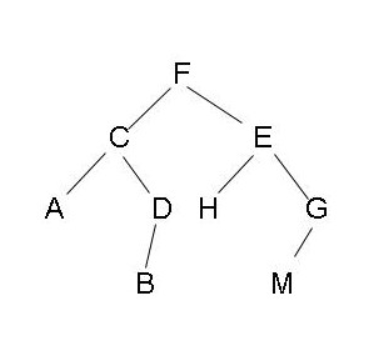2020/11/09

# 谈谈递归与树

## 递归

``````void f(){
f();
}
``````

``````void f(){
if (conditon){
return;
}
f();
}
``````

## 树## 两者的联系

1. 递归的基线条件就是二叉树叶子节点的判定条件
2. 对函数的递归调用就是对子树的访问（遍历）

## 应用

``````class Solution {
public List<Integer> inorderTraversal(TreeNode root) {
List<Integer> list = new ArrayList<>();
walk(root, list);
return list;
}
private void traversal(TreeNode root, List<Integer> list){
if (root == null) return;
traversal(root.left, list);
traversal(root.right, list);
}
}
``````

``````class Solution {
public TreeNode invertTree(TreeNode root) {
invertTree0(root);
return root;
}
private void invertTree0(TreeNode root){
if (root == null) return;

TreeNode t = root.left;
root.left = root.right;
root.right = t;

if (root.left !=null) invertTree0(root.left);
if (root.right !=null) invertTree0(root.right);
}
}
``````